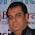## Thursday, August 15, 2013

### Filter a Revit Schedule for Whole Values

I saw an interesting question asked in the BIM + Revit MEP LinkedIn group the other day. The question was if it was possible to use wildcards in a schedule's filters (nope). But the real question was: would it be possible to filter a schedule so that it only displays values that are not whole numbers (integers).

It would be wonderful if I could provide an example using Autodesk® Revit® MEP's Elevation parameter, but alas, that built-in parameter is still not available for tagging or schedules.

However, here is the approach I used with a shared parameter used to report elevation. I am using three calculated values. I could get by with just one, but I like the idea of having one value to convert the elevation to a real number and one value to calculate the whole number, for QC purposes, and the third value to provide the actual filtering.

Here are the fields that I used:
1. Sparling Mounting Height: Shared parameter, Length, that reports the elevation of the element. How I wish I could use Elevation here!
2. Elevation As Number (can be hidden): Calculated value, Number, using this formula: (Sparling Mounting Height / 0' 1")
3. Whole Value (can be hidden): Calculated value, Number, using this formula: round(Elevation As Number)
4. Is Whole (can be hidden): Calculated value, Yes/No, using this formula: Elevation As Number = Whole Value

Note that the Round function does not work with lengths so it is neccessary to normalize the value from Sparling Mounting Height: (Sparling Mounting Height / 0' 1")

Update: Bruce Johnson pointed out that I could normalize using inches rather than feet and Revit converts the value correctly.

Because lengths are stored in Revit as decimal feet, the normalized value needs to be converted to inches before it is rounded: (Sparling Mounting Height / 1') * 12)

I round the calculated value of the elevation, in inches, normalized to a number to be used to test if the original value was a whole number.

Finally, we do the actual test to provide a Yes/No result that can be used to filter the schedule.

I also wish that I could resize the Calculated Value dialog so that it would be easier to document my schedules. However, I have no fix for that, other than adding it to the AUGI wishlist.

Autodesk, the Autodesk logo, AutoCAD, Navisworks, and Revit are registered trademarks or trademarks of Autodesk, Inc., and/or its subsidiaries and/or affiliates in the USA and/or other countries.

1.2.3.